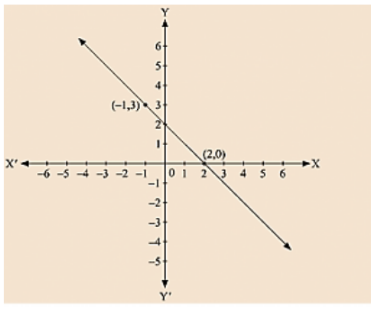# From the choices given below, choose the equation whose graph is given fig:

Question:

From the choices given below, choose the equation whose graph is given fig:

(i) y = x + 2

(ii) y = x – 2

(iii) y = - x + 2

(iv) x + 2y = 6

Solution:

We are given co-ordinates (-1, 3) and (2, 0) as the solution of one of the following equations.

We will substitute the value of both co-ordinates in each of the equation and find the equation which satisfies the given co-ordinates.(i) We are given, y=x+2 Substituting x = - 1 and y = 3,

We get 3 ≠ -1+ 2

L.H.S ≠ R.H.S

Substituting x = 2 and y = 0,

We get 0 ≠ 4

L.H.S ≠ R.H.S

Therefore, the given solution does not satisfy this equation.

(ii) We are given, y = x - 2

Substituting x = 1 and y = 3,

We get 3 = - 1 - 2

L.H.S ≠ R.H.S

Substituting x = 2 and y = 0, we get 0 = 0

L.H.S = R.H.S

Therefore, the given solutions does not completely satisfy this equation.

(iii) We are given, y = - x + 2

Substituting x = - 1 and y = 3,

We get 3 = - (-1) + 2

L.H.S = R.H.S

Substituting x = 2 and y = 0, we get 0 = - 2 + 2

0 = 0

L.H.S = R.H.S

Therefore, the given solutions satisfy this equation. Thus, it is the equation whose graph is given.